# MCAT Physical : Ohm's Law

## Example Questions

### Example Question #61 : Ap Physics 2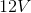battery supplies power to a circuit with a current of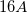. What is the resistance in this circuit?

Possible Answers: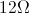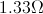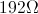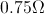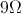Correct answer:Explanation:

To find resistance from voltage and current, we will need to use Ohm's law: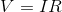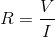We are given the voltage and current, allowing us to solve for the resistance.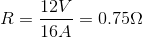### All MCAT Physical Resources